11.3 Probability of Independent Events

# Learning Objectives

• I can find the probability of at least one of two independent events occurring.
• I can find the probability of two independent events both occurring.
• I can find the probability of three independent events all occurring.
• I can use probability to predict the likelihood of real-life events.

# Introduction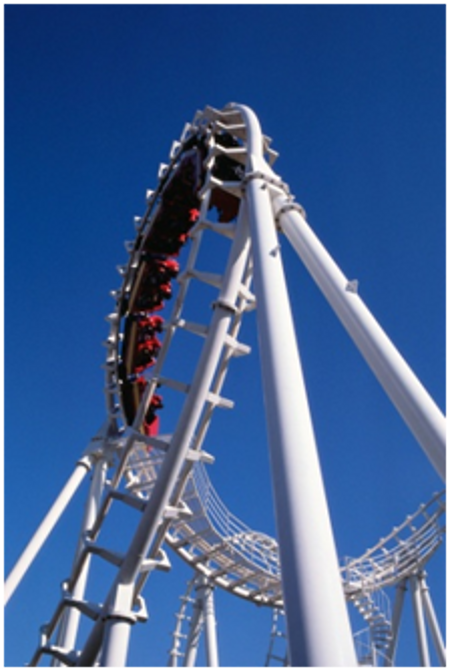The Amusement Park Survey

On the way back from Valleyfair, Mrs. Martinez gave her students a survey to fill out. She asked them to write down the rides that they enjoyed and to explain why they enjoyed them. All of the students had had a terrific time and riding on the coach bus to and from the amusement park had made everything wonderful!

Mrs. Martinez collected 25 surveys. While the bus traveled home, Mrs. Martinez reviewed the students’ answers.

Based on their responses, she could see that the students had really enjoyed this class trip. She noticed that 18 students had enjoyed the roller coaster and 12 students had enjoyed the bumper cars. These were the two most popular rides. She also noticed that some of the students had enjoyed both of these rides.

Mrs. Martinez wondered what the likelihood was that this would happen. What was the likelihood that a student would like both the roller coaster and the bumper cars?

Can you figure this out? Both of the events are independent of each other. Therefore, the probability of liking both rides is independent; one does not depend on the other. In this lesson you will learn how to calculate the probability of independent events. At the end of this lesson you will be able to figure out the likelihood of a student liking both the roller coaster and the bumper cars.

# Guided Learning

## Find the Probability of at Least One of Two Independent Events Occurring

When we think about probability, we think about the chances or the likelihood that something is going to happen. Sometimes, one event will happen and then a second event will happen right after that. Think about choosing a card from a deck of cards. We know that there are 52 cards in a deck, we can choose a card and there is a certain probability that it will be a red card. Then we can put the card back and choose again. The first outcome of choosing a card has nothing to do with the second outcome.

These outcomes are called independent events.

An independent event is an event that does not depend on another event to determine its outcome. When we have two independent events, one outcome does not impact the outcome of the second event. Choosing the card from the deck is a good example of an independent event as long as you replace the cards after each time your draw.

We can calculate the probability of an independent event occurring.

Let’s say that I have four tiles flipped face down on a table.On the side we can’t see are different images. Three of them have a sun and one of them has a four leaf clover.

What is the probability of selecting a sun on the first flip?

This is an independent event. Nothing else impacts the outcome except the flip. If there are three suns then we have a 3 out of 4 chance of flipping a sun.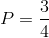We can also have two independent events. The events have nothing to do with each other, and yet we can calculate the probability of them occurring.

## Guided Practice

Practice calculating the likelihood of an independent event using a percent.

1. If 6 out of 24 students enjoy opera in a class, what is the probability that a student selected at random from the class will enjoy the opera?
2. If 4 out of 12 students like Math class best, what is the probability that a student selected at random from the class will enjoy Math best?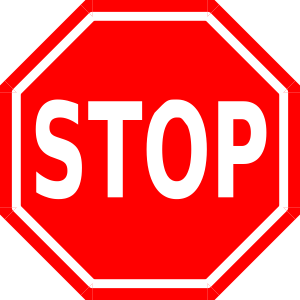Take a few minutes to discuss your answers with a friend. Resolve any differences between your answers.

Solutions

1.  P = 6/24 = ¼

2.  P = 4/12 = ⅓

## Find the Probability of Two Independent Events Both Occurring

How can we find the probability of two independent events both occurring? Well, first there are a few things that you need to know. In order for two events to be independent they can’t impact each other. Look at how that applies to this example.

### Example A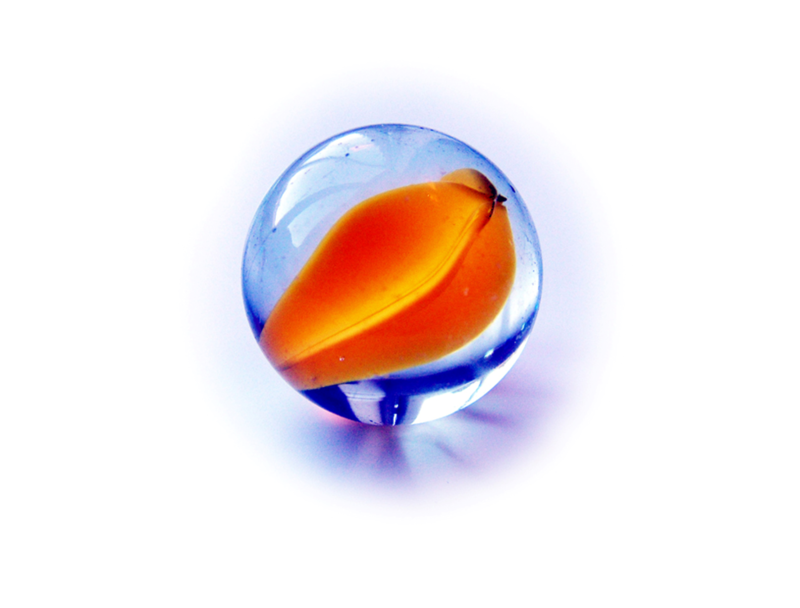There are 9 marbles in a bag. There are three blue, three green, and three orange. What is the probability of selecting one blue marble, then putting that one back and selecting one green marble?

First, notice that there are two independent events.

The first is to choose one blue marble.

The next is to choose one green marble.

Also notice that the first marble is put back into the bag before the next one is taken out; this is a key for independent events!

Now let’s think about the problem.

What is the probability of choosing a blue one first?

There are 9 marbles and 3 are blue.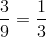What is the probability of choosing a green one next?

There are 9 marbles and 3 are green.To find the likelihood of these two independent events occurring, we multiply the two probabilities.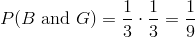Next, we can look at a real life example.### Example B

Out of 100 students at Trailview Elementary School, 50 enjoy swimming. Out of the same 100 students, 25 enjoy golf. What is the probability that a student would enjoy both swimming and golf?

To figure this out we have to figure out the probability of each independent event and then multiply them.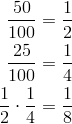There is a one out of eight chance that this will happen.

## Find the Probability of Three Independent Events Occurring

What about if we had three independent events? We would do the same operation. We find the likelihood of each event and then we multiply to the find the probability.

### Example C

There are 9 marbles in a bag. There are three blue, three green, and three orange. What is the probability of selecting one blue marble, then putting that one back and selecting one green marble, then putting that one back and selecting one orange marble?

Here we have the same work to do.

What is the probability of choosing a blue one first?

There are 9 marbles and 3 are blue.What is the probability of choosing a green one next?

There are 9 marbles and 3 are green.What is the probability of choosing an orange one next?

There are 9 marbles and 3 are orange.Now we figure out the likelihood of three independent events occurring by multiplying each probability.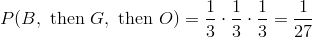There is a very small likelihood that this event will occur.

Now go back to the swimming and golf example.

### Example D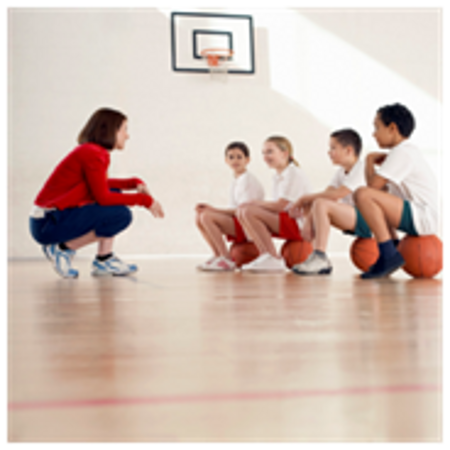Out of 100 students at Trailview Elementary School, 50 enjoy swimming. Out of the same 100 students, 25 enjoy golf. What is the probability that a student would enjoy both swimming and golf?

Let’s add to this problem that 40 out of 100 enjoy basketball.

What is the likelihood that a student would enjoy all three?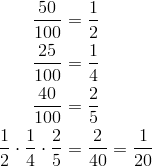There is a one out of 20 chance that a student would like all three.

We can look at this as a percent too and this will give us an idea of how small the likelihood is.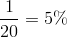## Real Life Example Completed

The Amusement Park Survey

Here is the original problem once again. Reread it and underline any important information.

On the way back from Valleyfair, Mrs. Martinez gave her students a survey to fill out. She asked them to write down the rides that they enjoyed and to explain why they enjoyed them. All of the students had had a terrific time and riding on the coach bus to and from the amusement park had made everything wonderful!

Mrs. Martinez collected 25 surveys. While the bus traveled home, Mrs. Martinez reviewed the students’ answers.

Based on their responses, she could see that the students had really enjoyed this class trip. She noticed that 18 students had enjoyed the roller coaster and 12 students had enjoyed the bumper cars. These were the two most popular rides. She also noticed that some of the students had enjoyed both of these rides.

Mrs. Martinez wondered what the likelihood was that this would happen. What was the likelihood that a student would like both the roller coaster and the bumper cars?

First, we have to figure out the probability of each of the independent events.

18 out of 25 students enjoyed the roller coaster.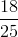12 out of 25 enjoyed the bumper cars.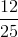This is a tricky problem because the numbers are larger. We can convert each fraction to a decimal and then multiply the decimals. After doing that, we can convert the decimal to a percent and we will be able to determine the probability.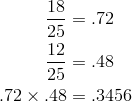We move the decimal point two places to the right and add a percent sign.

34.56 %

The probability that a student would have chosen both the roller coaster and the bumper cars is 34.56 or approximately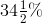.

# Review

• Calculate the probability of multiple events by multiplying the probability of each event together.

Probability

The probability that something will happen are the chances that something will happen. It can be written as a fraction, decimal, or percent.

Independent Event

An independent event is an event where the outcome of one event does not have anything to do with the outcome of another event. One does not alter or impact the other.

# Additional Resources

Compound Probability of Independent Events Video